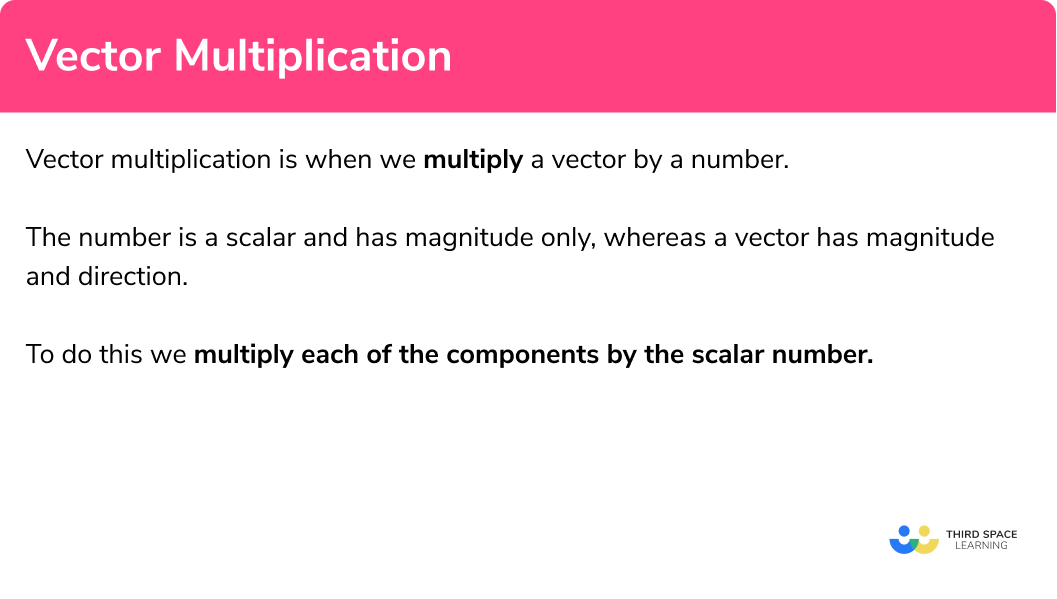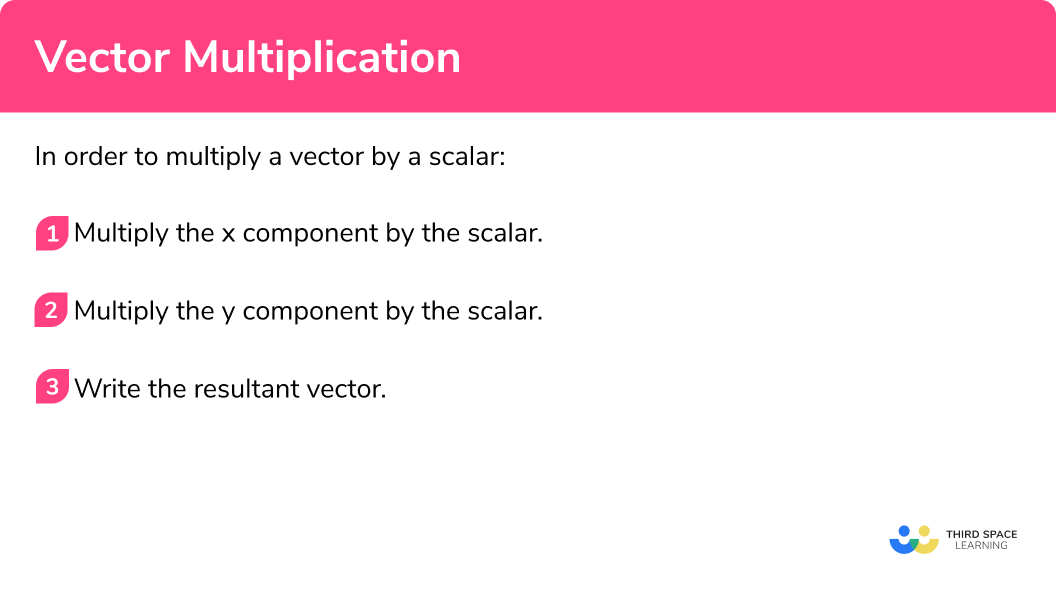GCSE Maths Geometry and Measure Vectors

Vector Multiplication

# Vector Multiplication

Here we will learn about vector multiplication, including scalar multiplication of a vector (multiplication of a vector by a number).

There are also vector worksheets based on Edexcel, AQA and OCR exam questions, along with further guidance on where to go next if you’re still stuck.

## What is vector multiplication?

Vector multiplication is when we multiply a vector by a number.  The number is a scalar and has magnitude only, whereas a vector has magnitude and direction.

To do this we multiply each of the components by the scalar number.

Here is an example:

Vector a is

$\textbf{a}= \left(\begin{array}{1} 2\\ 1\\ \end{array}\right)$

So multiplying the vector a by 3 would be

$3\textbf{a}= 3\left(\begin{array}{1} 2\\ 1\\ \end{array}\right) = \left(\begin{array}{1} 3\times2\\ 3\times 1\\ \end{array}\right) = \left(\begin{array}{1} 6\\ 3\\ \end{array}\right)$

This works because multiplication is repeated addition. Another way of thinking about multiplying the vector a by 3 would be

$3\textbf{a}=\textbf{a}+\textbf{a}+\textbf{a}= \left(\begin{array}{1} 2\\ 1\\ \end{array}\right) +\left(\begin{array}{1} 2\\ 1\\ \end{array}\right) +\left(\begin{array}{1} 2\\ 1\\ \end{array}\right) =\left(\begin{array}{1} 6\\ 3\\ \end{array}\right)$

We can see this on a diagram. You can see 3 lots of the x component and 3 lots of the y component.

The direction of the vector when it is multiplied by a scalar number is the same as the original vector, but the magnitude of the vector (also called the absolute value of the vector) has changed.

### What is vector multiplication?## How to multiply a vector

In order to multiply a vector by a scalar:

1. Multiply the x component by the scalar.
2. Multiply the y component by the scalar.
3. Write the resultant vector.

### How to multiply a vector## Related lessons on vectors

Vector multiplication is part of our series of lessons to support revision on vectors. You may find it helpful to start with the main vectors lesson for a summary of what to expect, or use the step by step guides below for further detail on individual topics. Other lessons in this series include:

## Vector multiplication examples

### Example 1: vector multiplication

Work out:

$2\left(\begin{array}{1} 4\\ 5\\ \end{array}\right)$

1. Multiply the x component by the scalar.

Multiply the scalar number by the top number

2\times4=8

2Multiply the y component by the scalar.

Multiply the scalar number by the bottom number

2\times5=10

3Write the resultant vector.

Write the two answers as a column vector

$2\left(\begin{array}{1} 4\\ 5\\ \end{array}\right) = \left(\begin{array}{1} 2\times4\\ 2\times5\\ \end{array}\right) = \left(\begin{array}{1} 8\\ 10\\ \end{array}\right)$

### Example 2: vector multiplication

Work out:

$4\left(\begin{array}{1} 8\\ 3\\ \end{array}\right)$

Multiply the scalar number by the top number

4\times8=32

Multiply the scalar number by the bottom number

4\times3=12

Write the two answers as a column vector

$4\left(\begin{array}{1} 8\\ 3\\ \end{array}\right) = \left(\begin{array}{1} 4\times8\\ 4\times3\\ \end{array}\right) = \left(\begin{array}{1} 32\\ 12\\ \end{array}\right)$

### Example 3: vector multiplication, decimal scalar

Work out:

$0.5\left(\begin{array}{1} 10\\ 8\\ \end{array}\right)$

Multiply the scalar number by the top number

0.5\times10=5

Multiply the scalar number by the bottom number

0.5\times8=4

Write the two answers as a column vector

$0.5\left(\begin{array}{1} 10\\ 8\\ \end{array}\right) = \left(\begin{array}{1} 0.5\times10\\ 0.5\times8\\ \end{array}\right) = \left(\begin{array}{1} 5\\ 4\\ \end{array}\right)$

### Example 4: vector multiplication, fractional scalar

Work out:

$\frac{1}{3}\left(\begin{array}{1} -6\\ 9\\ \end{array}\right)$

Multiply the scalar number by the top number

\frac{1}{3}\times-6=-2

Multiply the scalar number by the bottom number

\frac{1}{3}\times9=3

Write the two answers as a column vector

$\frac{1}{3}\left(\begin{array}{1} -6\\ 9\\ \end{array}\right) = \left(\begin{array}{1} \frac{1}{3}\times-6\\ \frac{1}{3}\times9\\ \end{array}\right) = \left(\begin{array}{1} -2\\ 3\\ \end{array}\right)$

### Example 5: vector multiplication

Work out:

$4\left(\begin{array}{1} -5\\ -2\\ \end{array}\right)$

Multiply the scalar number by the top number

4\times-5=-20

Multiply the scalar number by the bottom number

4\times-2=-8

Write the two answers as a column vector

$4\left(\begin{array}{1} -5\\ -2\\ \end{array}\right) = \left(\begin{array}{1} 4\times-5\\ 4\times-2\\ \end{array}\right) = \left(\begin{array}{1} -20\\ -8\\ \end{array}\right)$

### Example 6: vector multiplication, negative scalar

Work out:

$-3\left(\begin{array}{1} -2\\ 5\\ \end{array}\right)$

Multiply the scalar number by the top number

-3\times-2=6

Multiply the scalar number by the bottom number

-3\times5=-15

Write the two answers as a column vector

$-3\left(\begin{array}{1} -2\\ 5\\ \end{array}\right) = \left(\begin{array}{1} -3\times-2\\ -3\times5\\ \end{array}\right) = \left(\begin{array}{1} 6\\ -15\\ \end{array}\right)$

### Common misconceptions

• Make sure you multiply both components by the scalar number

Both the x component and the y component need to be multiplied by the scalar number.

• Column vectors only have 2 numbers within the brackets

Column vectors have the top number and the bottom number in the brackets. There is no need for any other punctuation marks such as commas or semicolons. There is no need for a line to separate the numbers.

### Practice vector multiplication questions

1. Work out 3\textbf{a} when

\textbf{a}= \begin{pmatrix} \; 2 \;\\ \; 4 \; \end{pmatrix}

\begin{pmatrix} \; 5 \;\\ \; 7 \; \end{pmatrix}\begin{pmatrix} \; 6 \;\\ \; 4 \; \end{pmatrix}\begin{pmatrix} \; 12 \;\\ \; 6 \; \end{pmatrix}\begin{pmatrix} \; 6 \;\\ \; 12 \; \end{pmatrix}3\textbf{a}= 3\begin{pmatrix} \; 2 \;\\ \; 4 \; \end{pmatrix} = \begin{pmatrix} \; 3\times 2 \;\\ \; 3\times4 \; \end{pmatrix} = \begin{pmatrix} \; 6 \;\\ \; 12 \; \end{pmatrix}

2. Work out 5\textbf{b} when

\textbf{b}= \begin{pmatrix} \; 1 \;\\ \; 3 \; \end{pmatrix}

\begin{pmatrix} \; 6 \;\\ \; 8 \; \end{pmatrix}\begin{pmatrix} \; 5 \;\\ \; 3 \; \end{pmatrix}\begin{pmatrix} \; 5 \;\\ \; 15 \; \end{pmatrix}\begin{pmatrix} \; 6 \;\\ \; 15 \; \end{pmatrix}5\textbf{b}= 5\begin{pmatrix} \; 1 \;\\ \; 3 \; \end{pmatrix} = \begin{pmatrix} \; 5\times 1 \;\\ \; 5\times3 \; \end{pmatrix} = \begin{pmatrix} \; 5 \;\\ \; 15 \; \end{pmatrix}

3. Work out 0.1\textbf{c} when

\textbf{c}= \begin{pmatrix} \; 20 \;\\ \; 30 \; \end{pmatrix}

\begin{pmatrix} \; 21 \;\\ \; 31 \; \end{pmatrix}\begin{pmatrix} \; 20.1 \;\\ \; 30.1 \; \end{pmatrix}\begin{pmatrix} \; 2 \;\\ \; 3 \; \end{pmatrix}\begin{pmatrix} \; 20 \;\\ \; 3 \; \end{pmatrix}0.1\textbf{c}= 0.1\begin{pmatrix} \; 20 \;\\ \; 30 \; \end{pmatrix} = \begin{pmatrix} \; 0.1\times 20 \;\\ \; 0.1\times30 \; \end{pmatrix} = \begin{pmatrix} \; 2 \;\\ \; 3 \; \end{pmatrix}

4. Work out \frac{2}{5}\textbf{a} when

\textbf{a}= \begin{pmatrix} \; -10 \;\\ \; 15 \; \end{pmatrix}

\begin{pmatrix} \; -4 \;\\ \; 3 \; \end{pmatrix}\begin{pmatrix} \; -4 \;\\ \; 6 \; \end{pmatrix}\begin{pmatrix} \; -2 \;\\ \; 3 \; \end{pmatrix}\begin{pmatrix} \; -10 \;\\ \; 6 \; \end{pmatrix}\frac{2}{5}\textbf{a}= \frac{2}{5}\begin{pmatrix} \; -10 \;\\ \; 15 \; \end{pmatrix} = \begin{pmatrix} \; \frac{2}{5}\times -10 \;\\ \; \frac{2}{5}\times15 \; \end{pmatrix} = \begin{pmatrix} \; -4 \;\\ \; 6 \; \end{pmatrix}

5. Work out 6\textbf{d} when

\textbf{d}= \begin{pmatrix} \; -2 \;\\ \; -3 \; \end{pmatrix}

\begin{pmatrix} \; 12 \;\\ \; 18 \; \end{pmatrix}\begin{pmatrix} \; 4 \;\\ \; 3 \; \end{pmatrix}\begin{pmatrix} \; -12 \;\\ \; -18 \; \end{pmatrix}\begin{pmatrix} \; -4 \;\\ \; -3 \; \end{pmatrix}6\textbf{d}= 6\begin{pmatrix} \; -2 \;\\ \; -3 \; \end{pmatrix} = \begin{pmatrix} \; 6\times -2 \;\\ \; 6\times -3 \; \end{pmatrix} = \begin{pmatrix} \; -12 \;\\ \; -18 \; \end{pmatrix}

6. Work out -3\textbf{f} when

\textbf{f}= \begin{pmatrix} \; -5 \;\\ \; -4 \; \end{pmatrix}

\begin{pmatrix} \; -15 \;\\ \; -12 \; \end{pmatrix}\begin{pmatrix} \; -2 \;\\ \; -1 \; \end{pmatrix}\begin{pmatrix} \; 15 \;\\ \; 12 \; \end{pmatrix}\begin{pmatrix} \; -15 \;\\ \; -4 \; \end{pmatrix}-3\textbf{f}= -3\begin{pmatrix} \; -5 \;\\ \; -4 \; \end{pmatrix} = \begin{pmatrix} \; -3\times -5 \;\\ \; -3\times -4 \; \end{pmatrix} = \begin{pmatrix} \; 15 \;\\ \; 12 \; \end{pmatrix}

### Vector multiplication GCSE questions

1. The vector \textbf{v} is

\textbf{v}= \begin{pmatrix} \; -3 \;\\ \; 5 \; \end{pmatrix}

Which of the vectors below is equivalent to 4\textbf{v} ?

(1 mark)

D

(1)

2. Vector \textbf{a} is

\textbf{a}= \begin{pmatrix} \; 3 \;\\ \; -6 \; \end{pmatrix}

Calculate 5\textbf{a}

(2 marks)

\begin{pmatrix} \; 15 \;\\ \; -30 \; \end{pmatrix}

For the x component (top number)

(1)

For the y component (bottom number)

(1)

3. Here is a vector multiplication

3 \begin{pmatrix} \; -4 \;\\ \; \text{b} \; \end{pmatrix} = \begin{pmatrix} \; \text{a} \;\\ \; 24 \; \end{pmatrix}

(a) Find the value of a .

(b) Find the value of b .

(2 marks)

(a)

a=-12

(1)

(b)

b=8

(1)

## Learning checklist

You have now learned how to:

• Multiply a vector by a scalar

## Did you know?

Not covered in GCSE: we can transpose a column vector to write it as a row vector (and vice versa). These look like co-ordinates, but do not have commas.

Vectors can also be extended into A Level Maths and Further Maths by learning how to multiply two vectors together using the dot product. This is also known as the scalar product of two vectors. It is possible to multiply vectors and this is known as a cross product. This is also known as the vector product of two vectors. The cross product is linked to the right hand rule.

We can also extend vectors into three dimensions. We can also look at the vector perpendicular to a plane to give the equation of the plane.

Column vectors are a simple example of matrices. In GCSE maths we have a single column. Matrices are studied in A Level Further Maths. The number of columns and rows will be more than 1 . Matrix multiplication can be studied along with finding the inverse of a matrix. We can also find the determinant of a matrix and go further and look into eigenvalues and eigenvectors.

If a vector has a magnitude of 1 , the vector is known as a unit vector.

## Still stuck?

Prepare your KS4 students for maths GCSEs success with Third Space Learning. Weekly online one to one GCSE maths revision lessons delivered by expert maths tutors.

Find out more about our GCSE maths tuition programme.